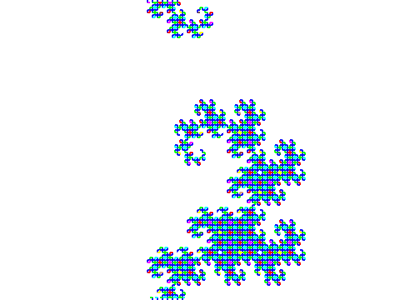# aUCBLogo Demos and Tests / curvesbe curves [singleshot false][FrameNr 0]

;
; You can generate any number of curves (Koch, Hilbert, Peano etc.)
; with this. By just defining a new expression. This is the
; same approach used in the 3D Hilbert except this is 2D.
;
; See the web page below for the different equations.
;
; Note: I used P for + and M for - in the equations.
;
; Note: In the Koch example F is both a graphics function
; (forward) and it's a recursive function. To deal with this
; I replaced all "F" with "KX" and let X do the recursion
; and K do the graphics (like F but only done at level 1).
;
;
; This one is still not quite right.
;

ht

demos=

{   [   cs pr [Koch]

DoFunction [X] [X K P X K M M X K P X K] [] 60 5 4

]

DoFunction [Y F] [Y F P X F P Y] [X F M Y F M X60 5 7

]

[   cs pr [Square]

DoFunction [F P X F P F P X F] [X F M F P F M X F P F P X F M F P F M X] [] 90 5 4

]

[   cs pr [Hilbert curve]

DoFunction [X] [P Y F M X F X M F Y P] [M X F P Y F Y P F X M90 5 5

]

[   cs pr [Dragon]

DoFunction [X] [X P Y F P] [M F X M Y90 5 12

]

}

ifelse singleshot

[   run demos.(FrameNr+1)

][   repeat count demos [run demos.repcount]

]

pu

home

pd

pr [Done]
end

to DoFunction :function_root :function_of_x :function_of_y :angle :siz :lev

if keyP [stop]

;   We are at the top level and we don't want X or Y to decrement :lev

make "lev :lev 1

; Run the base equation (all arguments are passed down implicity)

run :function_root

updateGraph

if not curves::singleshot

[   waitms 1000

]
end

to F

; :siz and :lev implicity passed from FX or FY or DoFunction

setpc :lev
;   tone 2*:lev 1      ;nice effect if you have time

fd :siz
end

to FX :siz :lev

; :function_of_x implicity passed from DoFunction

if :lev [run :function_of_x]
end

to FY :siz :lev

; :function_of_y implicity passed from DoFunction

if :lev [run :function_of_y]
end

to K

; :siz and :lev implicity passed from FX or FY or DoFunction

if (:lev == 1) [fd siz]
end

to M

; :angle implicity passed from DoFunction

lt angle
end

to P

; :angle implicity passed from DoFunction

rt angle
end

to X

; :siz and :lev implicity passed from FX or FY or DoFunction

fx siz lev-1
end

to Y

; :siz and :lev implicity passed from FX or FY or DoFunction

fy siz lev-1
end